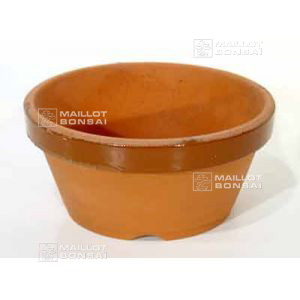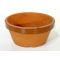##### The Japanese Bonsai specialist
Direct order Contact Help / Services Newsletter# Training flat bonsai pot n° 7

› Bonsai pots › Bonsai training potsref. : 4986

5,80

voluminous/heavy item extra shipping of 1,00

Available quantity : 8Order

###### Description

Diameter outside 21.5 cm. Interior diameter: 19.5 cm. Outside / interior height: 10.5/8.5 cm. To turn a tree into a bonsai you'll need this nursery pot, which is made in Japan and designed especially for this purpose. Featuring an enamelled flange and a large hole for drainage, this low training pot aids aeration and the development of surface nébari roots. For example, take a maple or a tree from nature and let the young sapling grow in this frost-resistant, fine earth pot. When it's ready you can repot it into an elegant bonsai pot.

#bonsai 6.6 #training 5.8 #this 4.4 #diameter 2.8 #interior 2.8 #outside 2.7 #into 2.4 #tree 2.4 #flat 2.4 #pots 2.4

Formule
(( ROUND((CHAR_LENGTH(b.article_nom)-CHAR_LENGTH(REPLACE(b.article_nom, 'bonsai', '')))/LENGTH('bonsai')) + ROUND((CHAR_LENGTH(b.article_description)-CHAR_LENGTH(REPLACE(b.article_description, 'bonsai', '')))/LENGTH('bonsai')) ) * 4.6) + (( ROUND((CHAR_LENGTH(b.article_nom)-CHAR_LENGTH(REPLACE(b.article_nom, 'this', '')))/LENGTH('this')) + ROUND((CHAR_LENGTH(b.article_description)-CHAR_LENGTH(REPLACE(b.article_description, 'this', '')))/LENGTH('this')) ) * 4.4) + (( ROUND((CHAR_LENGTH(b.article_nom)-CHAR_LENGTH(REPLACE(b.article_nom, 'training', '')))/LENGTH('training')) + ROUND((CHAR_LENGTH(b.article_description)-CHAR_LENGTH(REPLACE(b.article_description, 'training', '')))/LENGTH('training')) ) * 3.8) + (( ROUND((CHAR_LENGTH(b.article_nom)-CHAR_LENGTH(REPLACE(b.article_nom, 'diameter', '')))/LENGTH('diameter')) + ROUND((CHAR_LENGTH(b.article_description)-CHAR_LENGTH(REPLACE(b.article_description, 'diameter', '')))/LENGTH('diameter')) ) * 2.8) + (( ROUND((CHAR_LENGTH(b.article_nom)-CHAR_LENGTH(REPLACE(b.article_nom, 'interior', '')))/LENGTH('interior')) + ROUND((CHAR_LENGTH(b.article_description)-CHAR_LENGTH(REPLACE(b.article_description, 'interior', '')))/LENGTH('interior')) ) * 2.8) + (( ROUND((CHAR_LENGTH(b.article_nom)-CHAR_LENGTH(REPLACE(b.article_nom, 'outside', '')))/LENGTH('outside')) + ROUND((CHAR_LENGTH(b.article_description)-CHAR_LENGTH(REPLACE(b.article_description, 'outside', '')))/LENGTH('outside')) ) * 2.7) + (( ROUND((CHAR_LENGTH(b.article_nom)-CHAR_LENGTH(REPLACE(b.article_nom, 'flat', '')))/LENGTH('flat')) + ROUND((CHAR_LENGTH(b.article_description)-CHAR_LENGTH(REPLACE(b.article_description, 'flat', '')))/LENGTH('flat')) ) * 2.4) + (( ROUND((CHAR_LENGTH(b.article_nom)-CHAR_LENGTH(REPLACE(b.article_nom, 'into', '')))/LENGTH('into')) + ROUND((CHAR_LENGTH(b.article_description)-CHAR_LENGTH(REPLACE(b.article_description, 'into', '')))/LENGTH('into')) ) * 2.4) + (( ROUND((CHAR_LENGTH(b.article_nom)-CHAR_LENGTH(REPLACE(b.article_nom, 'tree', '')))/LENGTH('tree')) + ROUND((CHAR_LENGTH(b.article_description)-CHAR_LENGTH(REPLACE(b.article_description, 'tree', '')))/LENGTH('tree')) ) * 2.4) + (( ROUND((CHAR_LENGTH(b.article_nom)-CHAR_LENGTH(REPLACE(b.article_nom, 'development', '')))/LENGTH('development')) + ROUND((CHAR_LENGTH(b.article_description)-CHAR_LENGTH(REPLACE(b.article_description, 'development', '')))/LENGTH('development')) ) * 2.1)

## Secure payment## Delivery

Our logistic partners :